# Maharashtra Board Class 10 Maths Solutions Chapter 1 Linear Equations in Two Variables Practice Set 1.2

Maharashtra state Board 10th Standard Solutions Chapter 1 Linear Equations in two Variables – Here are all the MH Board solutions for 10th Standard maths Practice Set 1.2. This solution contains questions, answers, images, explanations of the complete Practice Set 1.2 titled Linear Equations in two Variables of maths taught in 10th Standard. If you are a student of 10th Standard who is using Maharashtra state Board Textbook to study maths, then you must come across Practice Set 1.2 Linear Equations in two Variables. After you have studied lesson, you must be looking for answers of its questions. Here you can get complete Maharashtra Board Solutions for 10th Standard maths Chapter 1 Linear Equations in two Variables in one place.

## Maharashtra State Board Class 10 Maths Solutions Chapter 1 Linear Equations in Two Variables Practice Set 1.2

Question 1.
Complete the following table to draw graph of the equations.
i. x + y = 3
ii. x – y = 4
i. x + y = 3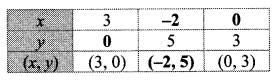ii. x – y = 4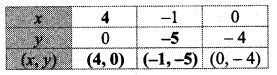Question 2.
Solve the following simultaneous equations graphically.
i. x + y = 6 ; x – y = 4
ii. x + y = 5 ; x – y = 3
iii. x + y = 0 ; 2x – y = 9
iv. 3x – y = 2 ; 2x – y = 3
v. 3x – 4y = -7 ; 5x – 2y = 0
vi. 2x – 3y = 4 ; 3y – x = 4
Solution:
i. The given simultaneous equations are
x + y = 6                                                                                                        x – y = 4
∴ y = 6 – x                                                                                                     ∴ y = x – 4The two lines intersect at point (5, 1).
∴ x = 5 and y = 1 is the solution of the simultaneous equations x + y = 6 and x – y = 4.

ii. The given simultaneous equations are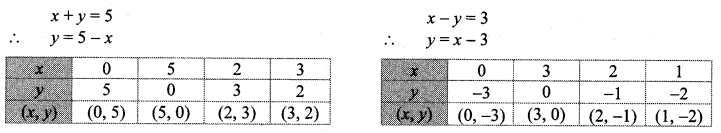The two lines intersect at point (4, 1).
∴ x = 4 and y = 1 is the solution of the simultaneous equations x+y = 5 and x – y = 3.

iii. The given simultaneous equations are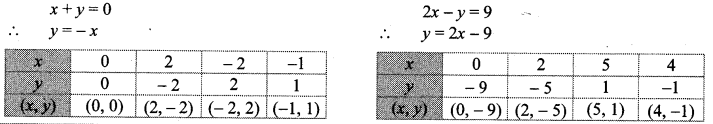The two lines intersect at point (3, -3).
∴ x = 3 and y = -3 is the solution of the simultaneous equations x + y = 0 and 2x – y = 9.

iv. The given simultaneous equations are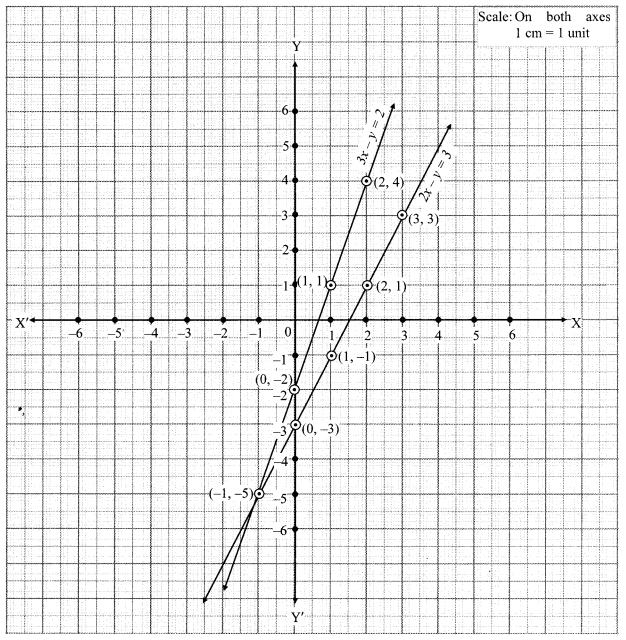The two lines intersect at point (-1, -5).
∴ x = -1 and y = -5 is the solution of the simultaneous equations 3x- y = 2 and 2x- y = 3.

v. The given simultaneous equations are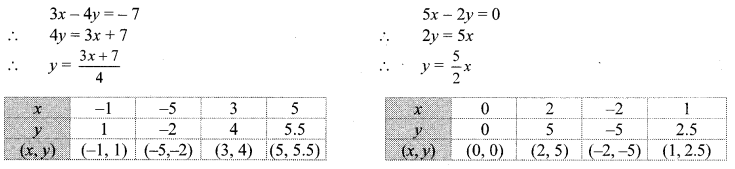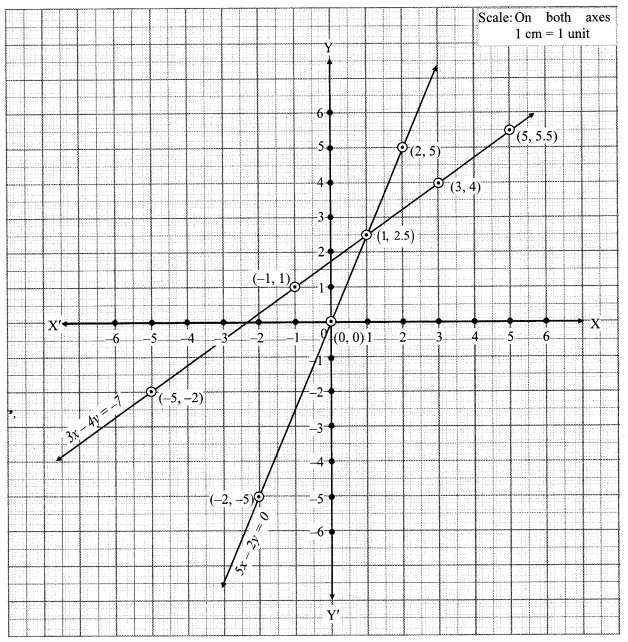The two lines intersect at point (1, 2.5).
∴ x = 1 and y = 2.5 is the solution of the simultaneous equations 3x – 4y = -7 and 5x – 2y = 0.

vi. The given simultaneous equations are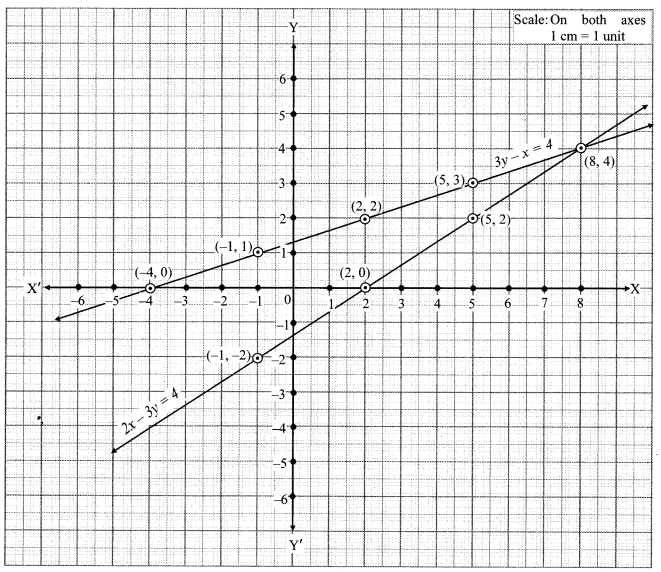The two lines intersect at point (8, 4).
∴ x = 8 and y = 4 is the solution of the simultaneous equations 2x – 3y = 4 and 3y – x = 4.

Question 1.
Solve the following simultaneous equations by graphical method. Complete the following tables to get ordered pairs.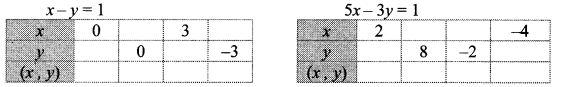i. Plot the above ordered pairs on the same co-ordinate plane.
ii. Draw graphs of the equations.
iii. Note the co-ordinates of the point of intersection of the two graphs. Write solution of these equations. (Textbook pg. no. 8)
Solution: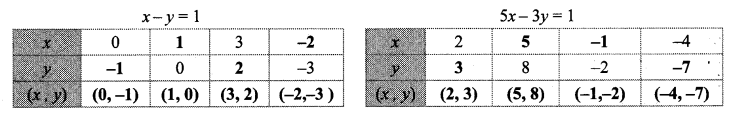The two lines intersect at point (-1, -2).
∴ (x , y) = (-1, -2) is the solution of the given simultaneous equations.

Question 1.
Solve the above equations by method of elimination. Check your solution with the solution obtained by graphical method. (Textbook pg. no. 8)
Solution:
The given simultaneous equations are
x – y = 1 …(i)
5x – 3y = 1 …(ii)
Multiplying equation (i) by 3, we get
3x – 3y = 3 …(iii)
Subtracting equation (iii) from (ii), we get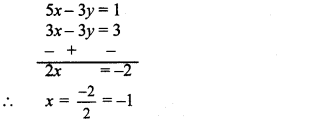Substituting x = -1 in equation (i), we get
-1 -y= 1
∴ -y = 1 + 1
∴ -y = 2
∴ y = -2
∴ (x,y) = (-1, -2) is the solution of the given simultaneous equations.
∴ The solution obtained by elimination method and by graphical method is the same.

Question 2.
The following table contains the values of x and y co-ordinates for ordered pairs to draw the graph of 5x – 3y = 1.i. Is it easy to plot these points?
ii. Which precaution is to be taken to find ordered pairs so that plotting of points becomes easy? (Textbook pg. no. 8)
Solution:
i. No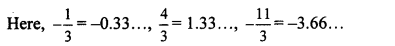The above numbers are non-terminating and recurring decimals.
∴ It is not easy to plot the given points.

ii. While finding ordered pairs, numbers should be selected in such a way that the co-ordinates obtained will be integers.

Question 3.
To solve simultaneous equations x + 2y = 4; 3x + 6y = 12 graphically, following are the ordered pairs.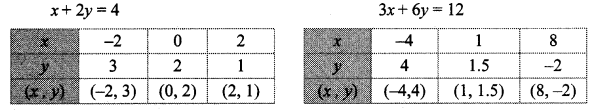Plotting the above ordered pairs, graph is drawn. Observe it and find answers of the following questions.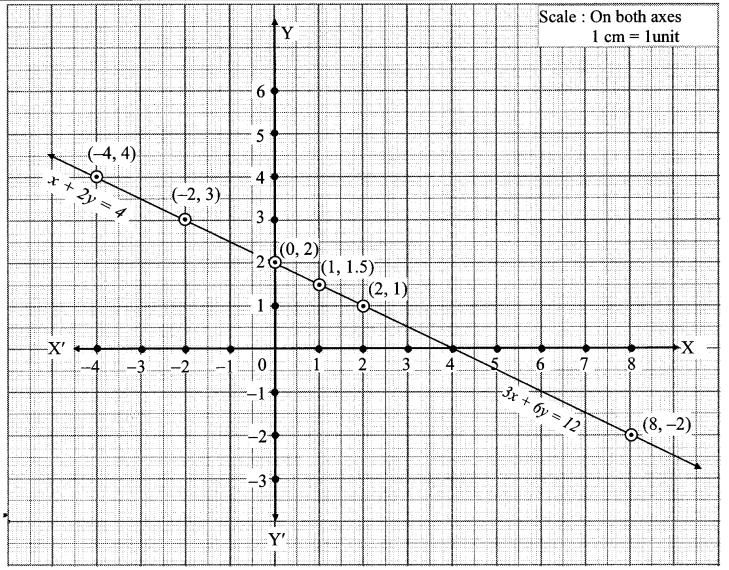i. Are the graphs of both the equations different or same?
ii. What are the solutions of the two equations x + 2y = 4 and 3x + 6y = 12? How many solutions are possible?
iii. What are the relations between coefficients of x, coefficients of y and constant terms in both the equations?
iv. What conclusion can you draw when two equations are given but the graph is only one line? (Textbook pg. no. 9)
Solution:
i. The graphs of both the equations are same.
ii. The solutions of the given equations are (-2, 3), (0, 2), (1, 1.5), etc.
∴ Infinite solutions are possible.
iii. Ratio of coefficients of x = $$\frac { 1 }{ 3 }$$
Ratio of coefficients of y = $$\frac { 2 }{ 6 }$$ = $$\frac { 1 }{ 3 }$$
Ratio of constant terms = $$\frac { 4 }{ 12 }$$ = $$\frac { 1 }{ 3 }$$
∴ Ratios of coefficients of x = ratio of coefficients of y = ratio of the constant terms
iv. When two equations are given but the graph is only one line, the equations will have infinite solutions.

Question 4.
Draw graphs of x- 2y = 4, 2x – 4y = 12 on the same co-ordinate plane. Observe it. Think of the relation between the coefficients of x, coefficients ofy and the constant terms and draw the inference. (Textbook pg. no. 10)
Solution: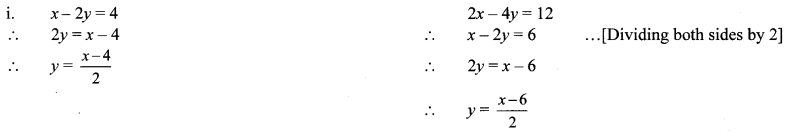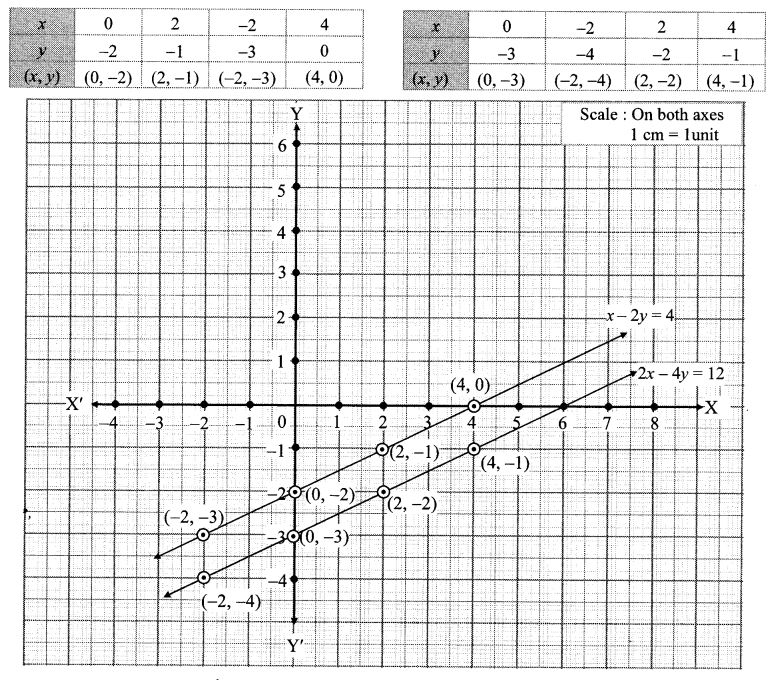ii. Ratio of coefficients of x =$$\frac { 1 }{ 2 }$$
Ratio of coefficients of y = $$\frac { -2 }{ -4 }$$ = $$\frac { 1 }{ 2 }$$
Ratio of constant terms = $$\frac { 4 }{ 12 }$$ = $$\frac { 1 }{ 3 }$$
∴ Ratio of coefficients of x = ratio of coefficients of y ratio of constant terms
iii. If ratio of coefficients of x = ratio of coefficients of y ≠ ratio of constant terms, then the graphs of the two equations will be parallel to each other.

Condition of consistency in Equations: